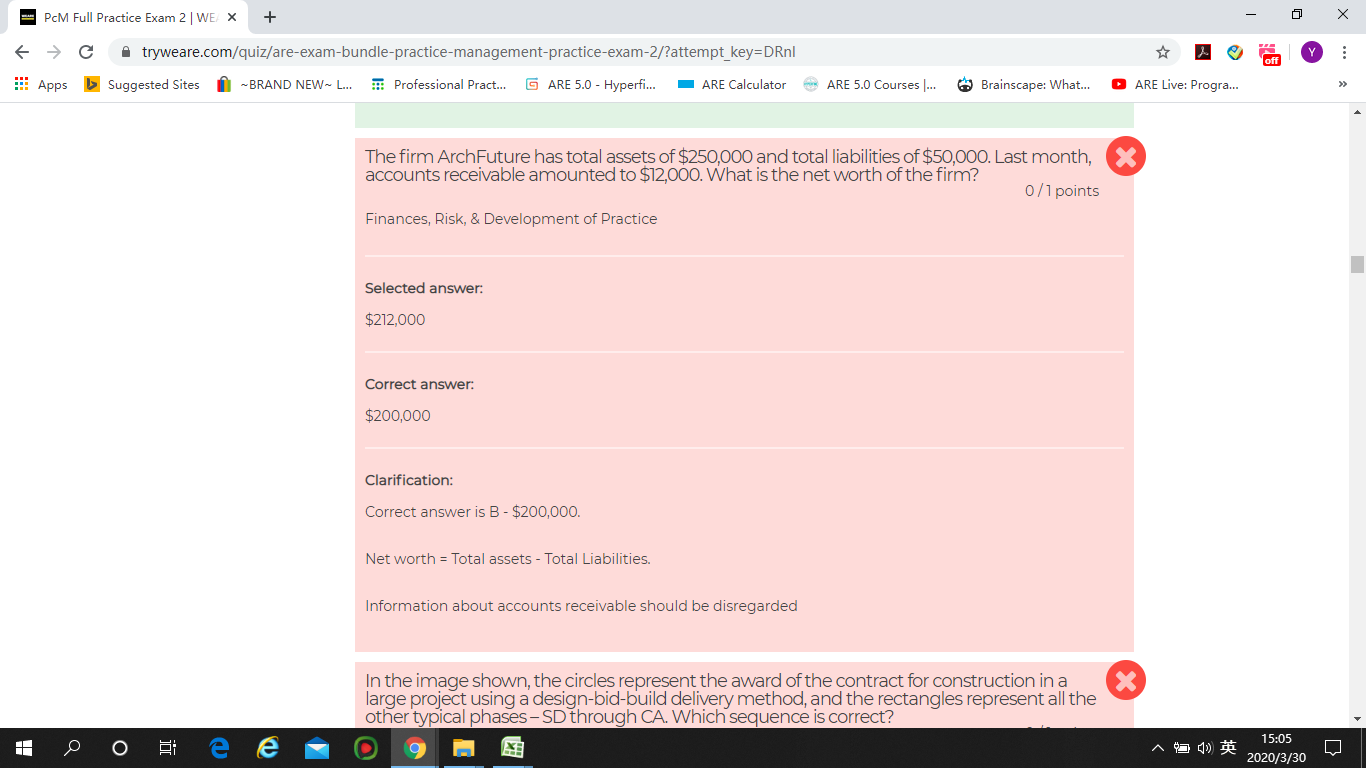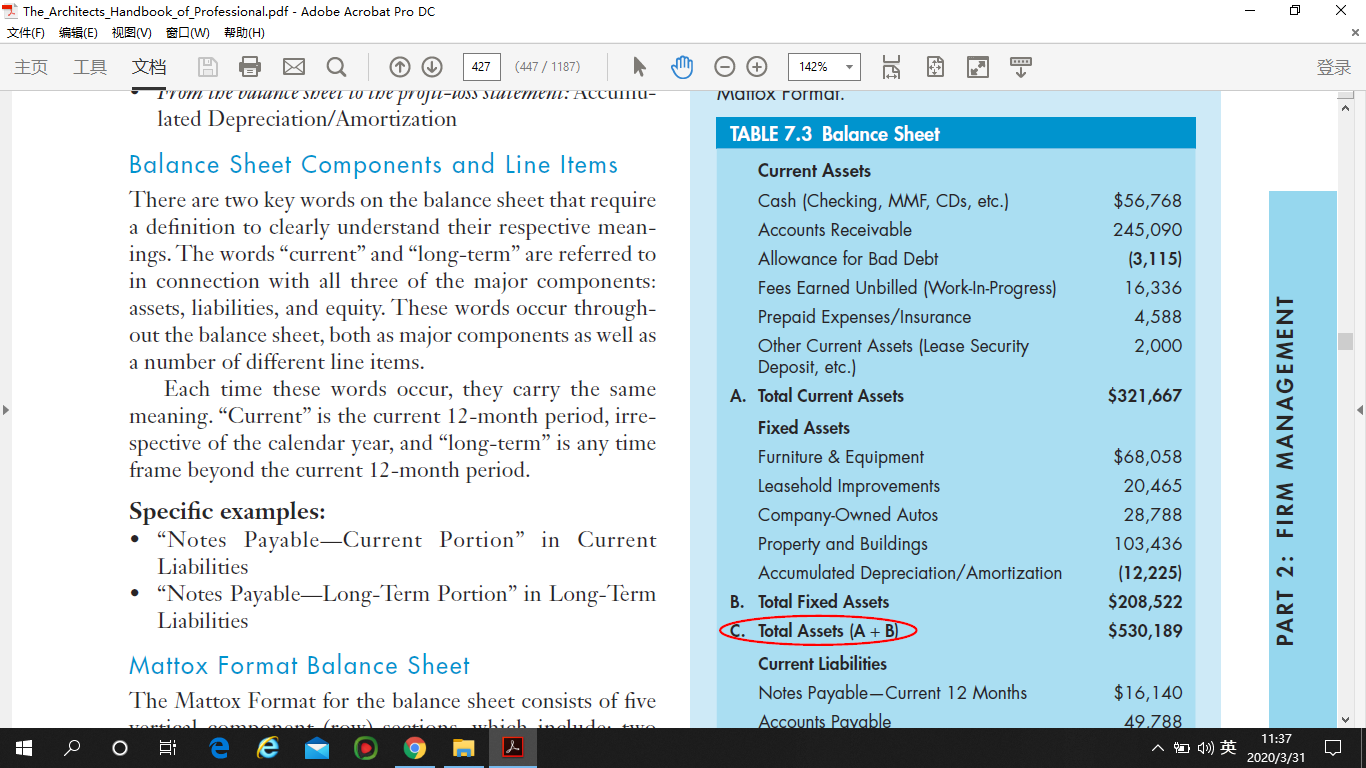•Account receivable and WIP are part from Current Assets, could you please share the WEARE question.

•Hi Haytham, here is the screenshot of WEARE question. Since account receivable is part of current assets, the right answer should be what I chose: \$212,000(\$250K plus \$12k minus \$50k). Please let me know.

Thanks,•Hi Yan, account receivable is part of Total Assets that's why it should be out of calculations. If the question mentioned specific assets (not total) then you can add account receivable to calculation of total assets.

•But, per AHPP, total asset = total current asset + total fixed asset. And account receivable is included in the total current asset. Therefore, when we calculate total asset, we should take account receivable into consideration.•Correct, but the information provided in the question was " Total Assets" which as per AHPP includes account receivable, so no need to double counting it.

•Ohhhhhh, now I understand it. The total asset has account receivable included. That is why. Thank you Haytham.

•You're welcome, I encourage you to go through Hyperfine course for financial management, it's very to understand all these from AHPP. Desk Crits also has a good summary.

•Thanks, me and my study buddy has finished Hyperfine financial part. Hyperfine is very helpful.

••